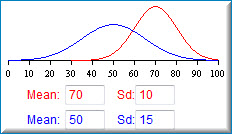# 7.4: Varieties Demonstration

•• Contributed by David Lane
• Associate Professor (Psychology, Statistics, and Management) at Rice University

Learning Objectives

• State the relationship between the mean and standard deviation of a distribution and its shape
• Be able to describe differences in distributions based on their shape and statistics

## Instructions

This demonstration allows you to change the mean and standard deviation of two normal distributions and observe the effects on the shapes of the distributions.When the demonstration begins, the red distribution has a mean of $$50$$ and a standard deviaton of $$10$$. The blue distribution has a mean of $$50$$ and a standard deviation of $$5$$. Notice how much more spread out the red distribution is than the blue distribution.

Choose different values for the two distributions and investigate their effects.

## Illustrated Instructions

The demonstration starts by displaying two curves both with a mean of $$50$$ and with standard deviations of $$10$$ and $$5$$ respectively. You can change the values for the mean and standard deviation for both curves.Figure $$\PageIndex{1}$$: Beginning curves for varieties demonstration

The screenshot below shows the distributions with different means and standard deviations. Note that the curves are on an axis that ranges from $$0 - 100$$, keep this in mind when changing the values of the mean and standard deviations as some values will not be displayed correctly.Figure $$\PageIndex{2}$$: Distributions with different means and standard deviations

## Contributor

• Online Statistics Education: A Multimedia Course of Study (http://onlinestatbook.com/). Project Leader: David M. Lane, Rice University.